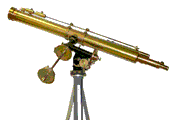## Research Papers in Physics and Astronomy## M. Eugene Rudd Publications

#### Date of this Version

11-1996

Published in Physical Review A Volume 54, Number 5, November 1996. Copyright © American Physical Society; used by permission.

#### Abstract

Energy-analyzed secondary electrons from H+ + Ar collisions at eight proton energies from 20 to 120 keV were detected in coincidence with recoil ions of charge states 1–3. The charge states were distinguished by time-of-flight measurements and the electron energies were analyzed by a newly designed electrostatic analyzer of the low-pass high-pass filter type which has a large (~1.45π steradian) solid angle acceptance. For each of nine electron energies from 10 to 160 eV, the ionizing events associated with each residual ion charge state were counted. The electron energy dependence of the charge-state ratios and the average charge determined from the experimental data were in satisfactory agreement with calculations made with a simple statistical model based on the assumption of independent interactions between the projectile and the ejected electrons. In this model the single-electron ionization probability is assumed to be given by P(b) = P(0)exp(-b/r0) where r0 is a characteristic length and b the impact parameter. Values of r0 and P(0) were determined by fitting the experimental data with this model. The charge-state ratios averaged over ejected electron energies agree with the data of DuBois et al.

COinS